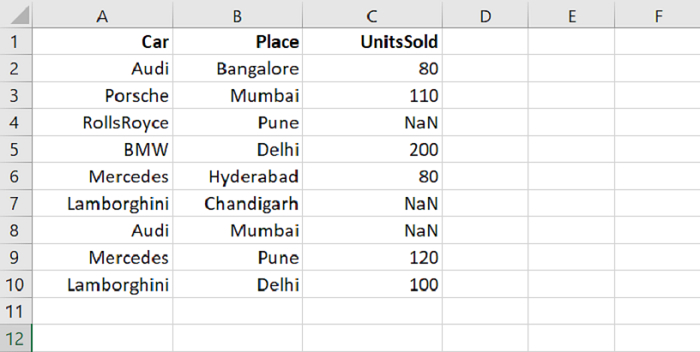# Python - How to drop the null rows from a Pandas DataFrame

PythonServer Side ProgrammingProgramming

To drop the null rows in a Pandas DataFrame, use the dropna() method. Let’s say the following is our CSV file with some NaN i.e. null values −Let us read the CSV file using read_csv(). Our CSV is on the Desktop −

dataFrame = pd.read_csv("C:\\Users\\amit_\\Desktop\\CarRecords.csv")

Remove the null values using dropna() −

dataFrame = dataFrame.dropna()


## Example

Following is the complete code −

import pandas as pd

print("DataFrame...\n",dataFrame)

# count the rows and columns in a DataFrame
print("\nNumber of rows and column in our DataFrame = ",dataFrame.shape)

dataFrame = dataFrame.dropna()
print("\nDataFrame after removing null values...\n",dataFrame)
print("\n(Updated) Number of rows and column in our DataFrame = ",dataFrame.shape)



## Output

This will produce the following output −

DataFrame...
Car       Place   UnitsSold
0         Audi   Bangalore        80.0
1      Porsche      Mumbai       110.0
2   RollsRoyce        Pune         NaN
3          BMW       Delhi       200.0
5  Lamborghini  Chandigarh         NaN
6         Audi      Mumbai         NaN
7     Mercedes        Pune       120.0
8  Lamborghini       Delhi       100.0

Number of rows and column in our DataFrame = (9, 3)

DataFrame after removing null values...
Car      Place   UnitsSold
0         Audi  Bangalore        80.0
1      Porsche     Mumbai       110.0
3          BMW      Delhi       200.0
(Updated) Number of rows and column in our DataFrame = (6, 3)